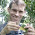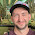## Sunday, March 17, 2013

### New, totally rewritten version of make.simmap; new phytools build

I just posted a totally rewritten version of the phytools function make.simmap for stochastic character mapping on trees. Rich Glor reported that there might be a bug in older versions of make.simmap because the posterior probabilities at internal nodes were sometimes different than the posterior probabilities computed using Jonathan Bollback's stand-alone Mac OS X program SIMMAP. That make.simmap and SIMMAP might give different results was not super concerning to me, because they do slightly different things. Specifically, 1) make.simmap is meant to sample the root state from the conditional distribution at the root, rather from the conditional distribution × the stationary distribution - as in equation (2) of Bollback (2006); and 2) make.simmap is meant to condition on the MLE of Q, the instantaneous transition matrix, rather than sampling transition rates among states from a user-specified prior distribution. [I believe I'm right about these two aspects of SIMMAP; please correct me if I have any details wrong.]

Anyway, Rich's email inspired me to take a close look at the code, which I'd written originally in mid-2011 and had been little modified since. I did find one bug - specifically, in computing probabilities to sample states from the multinomial, I had rescaled (incorrectly) by the maximum, rather than the sum, of the product of the conditional likelihoods × the transition probabilities - equation (3) in Bollback (2006). I.e.:
node.states[j,2]<-rstate(p/max(p))
in older versions of make.simmap (e.g., here), should have been:
node.states[j,2]<-rstate(p/sum(p))
This would not have mattered at all if I'd been smart and used the base function rmultinom, which automatically rescales probabilities internally to sum to 1.0 - but instead I used a custom phytools function rstate which (aside from also being computationally less efficient), did not. (rstate still exists, but now it calls rmultinom internally.)

The significance of this bug will depend on the specific case. In some empirical datasets that I ran, the effect on the posterior density (computed using densityMap) was small - but this should probably not be assumed.

In going closely over the code of make.simmap I also realized it was due a major overhaul. This is hardly surprising as I wrote the original version of this function "way back" in mid-2011 when I was only really starting to become adventurous in R after switching over from doing mostly everything in C. In re-writing make.simmap I've 'encapsulated' (so to speak) all the different steps of the mapping process into different internally called functions. I also removed the dependency of make.simmap on the 'ape' function ace for computing the conditional likelihoods - although in this case by borrowing liberally from the ace code (with attribution, of course) in a different internally called function, apeAce.

I also took advantage of the re-write to modify deviation 1) from Bollback's SIMMAP. Now the user has the option of assuming equal state frequencies, inputting arbitrary state frequencies, or estimating frequencies by numerically solving for the stationary distribution conditioned on Q. By multiplying our conditional likelihoods at the root by the stationary distribution, we are basically putting a prior on the root state that is equal to the stationary distribution implied by the fitted model. I'm not totally sure whether we want to do this or not. Feedback welcome.

One interesting case of user-defined state frequencies is the practice of setting the frequency at 1.0 for one state and 0.0 for the others. What this will result in is a set of stochastic maps conditioning on a particular value (the one with probability 1.0) at the root node of the tree - which might be interesting for some problems.

For make.simmap(...,message=TRUE) (the default), the function will now also spit back the value for the transition matrix, Q and the prior on the state frequencies at the root (π) used during simulation.

Finally, this updated version of make.simmap runs about 30-40% faster than the prior version (even with the specific bug-fix identified above), which is not trivial.

I also built a new version of phytools, phytools 0.2-27, which can be downloaded and installed from source in the usual way.

Here's a quick demo of use of make.simmap. [Note that the input matrix Q for simulation in sim.history is the transpose of the printed matrix Q in make.simmap. In the literature on molecular evolution models and Markov chains in general there is some discrepancy about whether the rows or columns should sum to 0.0 by convention (the only difference being whether we need to post- or pre-multiply eQt, respectively, by π(t) to get π(t+1)).]

> require(phytools)
> packageVersion("phytools")
 ‘0.2.27’
> tree<-pbtree(n=200,scale=1)
> Q<-matrix(c(-1,1,1,-1),2,2)
> rownames(Q)<-colnames(Q)<-letters[1:nrow(Q)]
> tree<-sim.history(tree,Q,anc="a")
> mtrees<-make.simmap(tree,tree\$states,model="ER", nsim=100,pi="estimated")
make.simmap is sampling character histories conditioned on the transition matrix
Q =
a          b
a -0.7876848  0.7876848
b  0.7876848 -0.7876848
(estimated using likelihood);
and state frequencies (used to sample the root node following Bollback 2006)
pi =
a   b
0.5 0.5
> cols<-setNames(c("blue","red"),letters[1:nrow(Q)])
> plotSimmap(mtrees[],cols,pts=F,ftype="off")
Now let's compute the state frequencies from the stochastic maps for each internal node. These are our posterior probabilities for nodes:
> # function to compute the states
> foo<-function(x){
+ y<-sapply(x\$maps,function(x) names(x))
+ names(y)<-x\$edge[,1]
+ y<-y[as.character(length(x\$tip)+1:x\$Nnode)]
+ return(y)
+ }
>
> AA<-sapply(mtrees,foo)
> piesA<-t(apply(AA,1,function(x,levels,Nsim) summary(factor(x,levels))/Nsim,levels=letters[1:3], Nsim=100))
> plot(tree,no.margin=TRUE,show.tip.label=F)
> nodelabels(pie=piesA,cex=0.6,piecol=cols)

Finally, let's compare to the generating map:
> # first compute the number of changes of each type
> XX<-countSimmap(mtrees,message=FALSE)
> colMeans(XX)
N   a,a   a,b   b,a   b,b
28.65  0.00 10.88 17.77  0.00
> # compare to the true history
> countSimmap(tree,message=FALSE)
\$N
 27
\$Tr
a  b
a  0 11
b 16  0
> # now compute the mean overlap with the true history
> xx<-sapply(mtrees,map.overlap,tree)
> mean(xx)
 0.9144295

I think that we can be pretty confident that make.simmap is doing what it's supposed to do; however please keep in mind that this version is brand new and has not been thoroughly tested. And, as Rich showed in pointing out this potential error to me, it is always wise to look behind the curtain - if possible - and don't hesitate to report any bugs, issues, or questionable results to me ASAP. I'll do whatever I can to address them.

#### 16 comments:

1.If anyone is interested in comparing make.simmap to SIMMAP, they should check out the phytools function export.as.xml which will create a SIMMAP input file.

In my very non-scientific comparison yesterday I found that SIMMAP, under the default conditions for morphology, sampled the same posterior probabilities at nodes as make.simmap - but that the number of changes in SIMMAP were too large (i.e., the 95% distribution did not include the generating number of changes). This might be just a matter of tuning the priors, or it might reflect something deeper. At this point, I just don't know.

2.Hy Liam,

thank you for making stochastic mapping available for R users.
Buy unfortunatelly I'm not getting it. I'll obtain the Q matrix as the output of make.simmap (and to use it in sim.history I have to transpose of the printed matrix Q of make.simmap), right? But when I try to run make.simmap I get the following message: "subscript out of bounds". I don't know what I'm doing wrong. The tree is in phylo format and I'm using SYM model. Can you please give me and example of the vector X ("a vector containing the tip states for a discretely valued character") so I can compare with mine? It is a simple vector like x=c("1","1","0","1")? One more question, how can I indicate missing information on the tip states?

1.Hi Elisa.

Yes, just a vector - but the names attribute must be the species names in the tree (in any order).

If you have uncertain, you can use a prior probability distribution on the tip states. This is described here. Basically, x becomes a matrix with n rows and m columns for n taxa and m values for the trait. Each element in the prior probability, Pr(i,j), of the ith taxon being in the jth state. In this case, row names should be tip labels and column names should be state names.

Let me know if this is what you needed to know. Liam

2.Hi Liam,

I have the same question as Elisa, but I'm not quite clear on your answer. Can you explain what you mean by "the names attribute?" I provided a simple vector like the example Elisa has above and I'm receiving the same error message. Thanks!

3.Hello, hope this question goes in the right place ;) Am I right that from describe.simmap I can get the transition rates simply by dividing the numbers of different transitions by the total number of all transitions? Sorry if this is silly but I'm still relatively new to comparative stuff and wan't just find similarities between phytools output and BayesTraits which I used so far.

4.Is there a way to fix specific transition rates in Q while allowing all others to be estimated at their most likely values?

5.This comment has been removed by the author.

6.Hi Liam,

Thanks for the detailed explanation. In terms of counting how many times a given transformation (e.g. 0->1) has occured, I was wondering if there is a way to generate a histogram if probability distributions for the number of changes based on the joint reconstruction.

I have used countSimmap, which returns an integer value for the number of changes, but I'm not sure how this number is calculated from the probability distributions for each node. You mention the possibility of generating histograms in your "Lecture_5.1" on ancestral state reconstructions, but I can't figure out a way of generating such an output.

Thanks a lot in advance!

7.Hi Liam,

I am working on an example to give to a class, I am using this format for trait data (as I previously used)

Species 1
Species 2

and reading it through

> dietario <- read.table("fruti.txt", row.names = 1)

Then I converted this with the following

> XXX <- dietario[,1]
> names(XXX) <- row.names(dietario)
> XXX -> dietas

Then I got this error

> make.simmap(arbol, dietas)
Error in xx[tree\$tip.label, ] : subscript out of bounds

The "arbol" and data had the same taxa

Thanks for your help!

1.Hi Andrés, did you solve this problem? I'm having the same one.

2.Sorry I couldn't make it work with that toy example and summarized the info in another way since I was in a rush for preparing that material.

3.Hi flashton. If you email me your R workspace and the specific command generating the error, I would be happy to try to get to the bottom of it. - Liam

4.Hi, it was resolved? I'm having the same error but with other functions.
Thanks

5.Hi Andrés,I geted the same error with other data, in my case that error was a difference on the names of the terminals coming from the tree and those from the data. I am not an exprt on R but to compare the names I extracted to a variable (M) the names of the tree with the tip.tree (M<-mcctree[["tip.label"]])function and then made a matrix with (M<-data.matrix(M)). Then I compared the data of M (the names) with those that comes from the data on a spreadsheet. I hope this helps. Best regards. Luis

6.Thank Luis, yes, it is usually some fine detail in the " or some inadverted typo.

8.Hello, I am having the same error, but I am pretty sure it is not due to incorrect matching of tip labels and species labels in my data, because i have removed all species in the data that do not match the tree\$tip.labels. the error I receive is
"Error in xx[tree\$tip.label, ] : subscript out of bounds"

I am happy to provide more information if needed.

Note: due to the very large amount of spam, all comments are now automatically submitted for moderation.